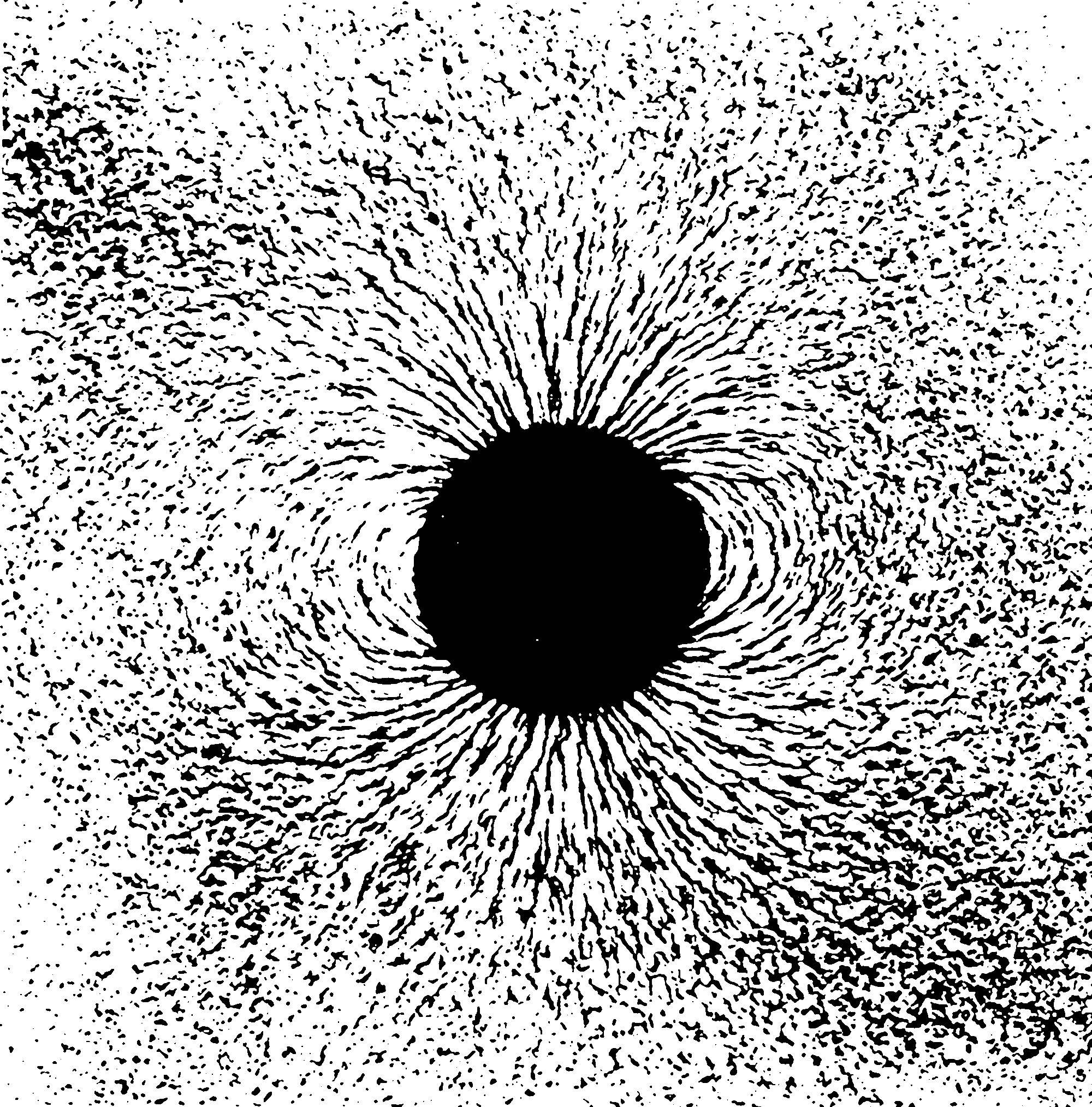# Causal inference in the continuous limit

Causality on continuous index spaces, and, which turns out to be related, equilibrium/feedback dynamics. Placeholder.Bongers and Mooij (2018):

Uncertainty and random fluctuations are a very common feature of real dynamical systems. For example, most physical, financial, biochemical and engineering systems are subjected to time-varying external or internal random disturbances. These complex disturbances and their associated responses are most naturally described in terms of stochastic processes. A more realistic formulation of a dynamical system in terms of differential equations should involve such stochastic processes. This led to the fields of stochastic and random differential equations, where the latter deals with processes that are sufficiently regular. Random differential equations (RDEs) provide the most natural extension of ordinary differential equations to the stochastic setting and have been widely accepted as an important mathematical tool in modeling…

Over the years, several attempts have been made to interpret these structural causal models that include cyclic causal relationships. They can be derived from an underlying discrete-time or continuous-time dynamical system. All these methods assume that the dynamical system under consideration converges to a single static equilibrium… These assumptions give rise to a more parsimonious description of the causal relationships of the equilibrium states and ignore the complicated but decaying transient dynamics of the dynamical system. The assumption that the system has to equilibrate to a single static equilibrium is rather strong and limits the applicability of the theory, as many dynamical systems have multiple equilibrium states.

In this paper, we relax this condition and capture, under certain convergence assumptions, every random equilibrium state of the RDE in an SCM. Conversely, we show that under suitable conditions, every solution of the SCM corresponds to a sample-path solution of the RDE. Intuitively, the idea is that in the limit when time tends to infinity the random differential equations converge exactly to the structural equations of the SCM.

“RDEs” seem to be stochastic differential equations with differentiable sample paths.

### No comments yet. Why not leave one?

GitHub-flavored Markdown & a sane subset of HTML is supported.